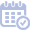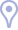# Talks

Exploiting Statistical Dependencies in Sparse RepresentationsJanuary 7th, 2011Invited talk in the SMALL workshop on Sparse Dictionary Learning.

In the commonly used sparse representation modeling, the atoms are assumed to be independent of each other when forming the signal. In this talk we shall introduce a statistical model called Boltzman Machine (BM) that enables such dependencies to be taken into account. Adopting a Bayesian point of view, we first treat the pursuit problem – given a signal, and assuming that the model parameters and the dictionary are known, find its sparse representation. We derive the exact MAP estimation, and show that just like in the independent case, this leads to an exponential search problem. We derive two algorithms for its evaluation: a greedy approximation approach for the general case, and an exact estimation that corresponds to a unitary dictionary and banded interaction matrix. We also consider the estimation of the model parameters, learning these parameters directly from training data. We show that given the signals’ representations, this problem can be posed as a convex optimization task by using the Maximum Pseudo-Likelihood (MPL).This is a joint work with Tomer Faktor and Yonina Eldar (EE - Technion).